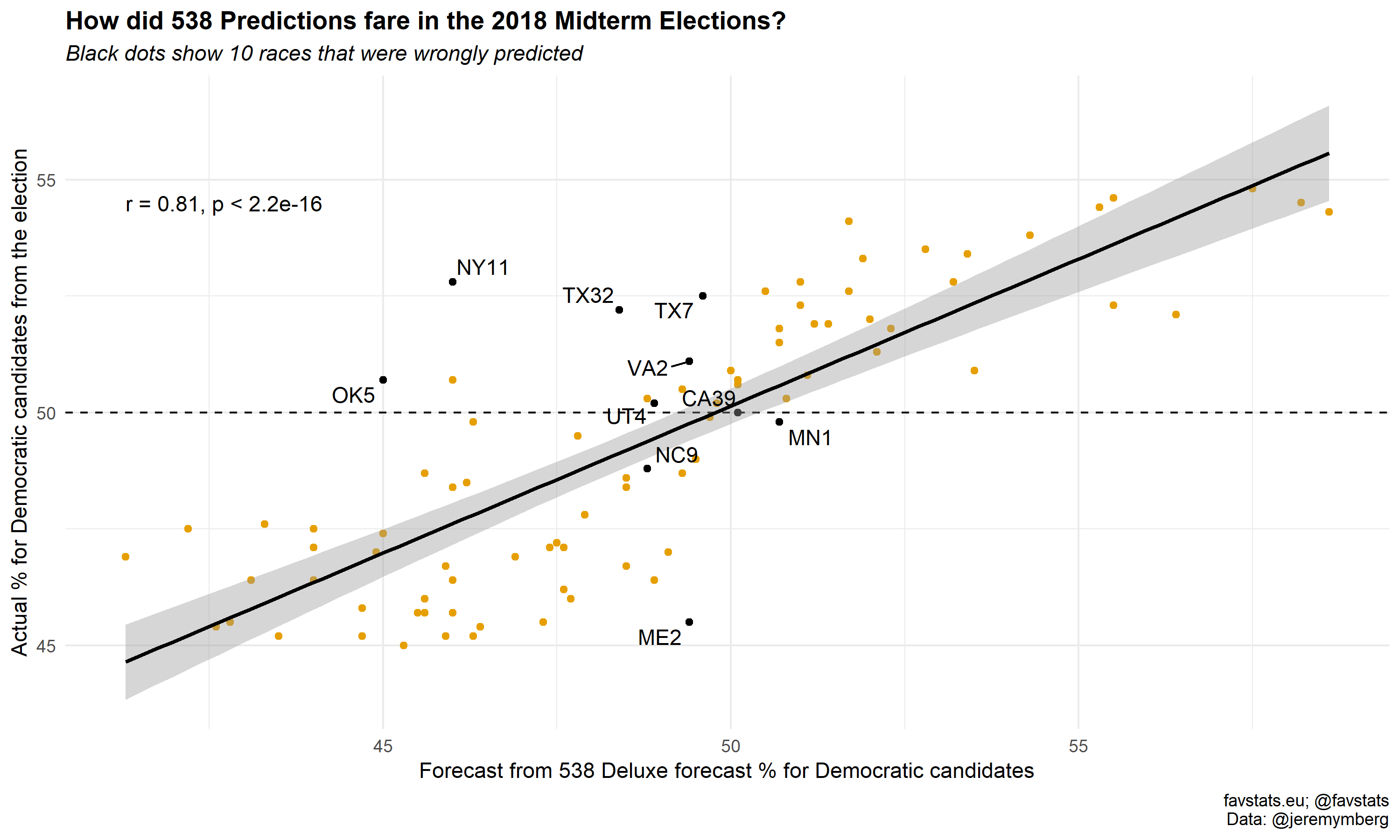Forecast from 538 Deluxe forecast

# 538 Predictions for the 2018 Midterm Elections

## Packages

# Install these packages if you don't have them yet
# if (!require("pacman")) install.packages("pacman")
# devtools::install_github("favstats/tidytemplate")

pacman::p_load(tidyverse, tidytemplate, janitor, ggthemes, ggpubr, rvest, ggrepel)


csv_link <- "https://blogs.sciencemag.org/sciencehound/wp-content/uploads/sites/5/2018/11/Congressional-election-results-and-forecasts.csv"

clean_names() %>%
mutate(close = ifelse(election_results <= 55 & election_results >= 45, "Close", "Safe")) %>%
left_join(tibble(state = state.name, abbr =state.abb)) %>%
mutate(distr_abbr = paste0(abbr, district))

pred_dat

## # A tibble: 435 x 14
##    state district democratic_cand~ republican_cand~ election_results
##    <chr>    <int> <chr>            <chr>                       <dbl>
##  1 Alab~        1 Kennedy          Byrne                        36.7
##  2 Alab~        2 Isner            Roby                         38.5
##  3 Alab~        3 Hagan            Rogers                       36.2
##  4 Alab~        4 Auman            Aderholt                     20.1
##  5 Alab~        5 Joffrion         Brooks                       38.9
##  6 Alab~        6 Kline            Palmer                       30.7
##  7 Alab~        7 Sewell           -                           100
##  8 Alas~        1 Glavin           Young                        46
##  9 Ariz~        1 O'Halleran       Rogers                       53.8
## 10 Ariz~        2 Kirkpatrick      Peterson                     54.4
## # ... with 425 more rows, and 9 more variables: predicted_lite <dbl>,
## #   predicted_classic <dbl>, predicted_deluxe <dbl>,
## #   predicted_probability_deluxe <dbl>, correct_deluxe <int>,
## #   democratic_win <int>, close <chr>, abbr <chr>, distr_abbr <chr>


## How did 538 Predictions fare in the 2018 Midterm Elections?

text_dat <- pred_dat %>%
count(correct_deluxe) %>%
rename(incorrect = 0, correct = 1) %>%
mutate(total = (incorrect + correct)) %>%
mutate(perc_correct = round(((correct / total)*100), 2)) %>%
mutate(label = glue::glue("538 predicted {correct} out of {total} races correctly ({perc_correct}%)"))

pred_dat %>%
mutate(correct_deluxe = as.factor(correct_deluxe)) %>%
ggplot(aes(predicted_deluxe, election_results)) +
geom_point(aes(color = correct_deluxe)) +
theme_minimal() +
scale_color_colorblind() +
geom_smooth(method = "lm", color = "black", alpha = 0.4) +
theme(legend.position = "bottom") +
geom_text(data = text_dat, aes(x = 22, y = 97, label = label)) +
labs(title = "How did 538 Predictions fare in the 2018 Midterm Elections?",
x="Forecast from 538 Deluxe forecast % for Democratic candidates",
y="Actual % for Democratic candidates from the election",
subtitle = "Black dots show 10 races that were wrongly predicted",
caption = "favstats.eu; @favstats\nData: @jeremymberg")  +
guides(color = F)  +
theme(plot.title = element_text(size = 13, face = "bold"),
plot.subtitle = element_text(size = 11, face = "italic"))ggsave_it(pred1, width = 10, height = 6)


## How did 538 Predictions fare in the 2018 Midterm Elections? - Close Races

pred_dat %>%
ggplot(aes(predicted_deluxe, election_results)) +
geom_point(aes(color = close)) +
theme_minimal() +
scale_color_colorblind() +
geom_hline(yintercept = 55, linetype = "dashed") +
geom_hline(yintercept = 45, linetype = "dashed") +
geom_smooth(method = "lm", color = "black", alpha = 0.4) +
theme(legend.position = "bottom") +
stat_cor() +
labs(title = "How did 538 Predictions fare in the 2018 Midterm Elections?",
x="Forecast from 538 Deluxe forecast % for Democratic candidates",
y="Actual % for Democratic candidates from the election",
subtitle = "Black dots show 89 races that were close",
caption = "favstats.eu; @favstats\nData: @jeremymberg")  +
guides(color = F)  +
theme(plot.title = element_text(size = 13, face = "bold"),
plot.subtitle = element_text(size = 11, face = "italic"))ggsave_it(pred2, width = 10, height = 6)


## How did 538 Predictions fare in the 2018 Midterm Elections? - Close Races II

pred_dat %>%
mutate(correct_deluxe = as.factor(correct_deluxe)) %>%
filter(close == "Close") %>%
ggplot(aes(predicted_deluxe, election_results)) +
geom_point(aes(color = correct_deluxe)) +
theme_minimal() +
geom_hline(yintercept = 50, linetype = "dashed") +
scale_color_colorblind() +
scale_y_continuous(breaks = c(45, 50, 55)) +
geom_smooth(method = "lm", color = "black", alpha = 0.4) +
geom_text_repel(data = pred_dat %>% filter(correct_deluxe == 0), aes(label = distr_abbr)) +
theme(legend.position = "bottom") +
stat_cor() +
labs(title = "How did 538 Predictions fare in the 2018 Midterm Elections?",
x="Forecast from 538 Deluxe forecast % for Democratic candidates",
y="Actual % for Democratic candidates from the election",
subtitle = "Black dots show 10 races that were wrongly predicted",
caption = "favstats.eu; @favstats\nData: @jeremymberg")  +
guides(color = F)  +
theme(plot.title = element_text(size = 13, face = "bold"),
plot.subtitle = element_text(size = 11, face = "italic"))ggsave_it(pred3, width = 10, height = 6)


## How did 538 Predictions fare in the 2018 Midterm Elections? - Prediction

hist_dat <- pred_dat %>%
mutate(diff = abs(election_results - predicted_deluxe)) %>%
arrange(desc(diff))

difference <- mean(hist_dat\$diff) %>% round(2)

hist_dat %>%
ggplot(aes(diff)) +
theme_minimal() +
geom_histogram(alpha = 0.75) +
geom_vline(xintercept = difference, linetype = "dashed") +
geom_text(aes(x = difference + .5, y = 62, label = difference)) +
labs(title = "How did 538 Predictions fare in the 2018 Midterm Elections?",
x="Absolute Difference between 538 Forecast and Results in %",
y="Frequency",
caption = "favstats.eu; @favstats\nData: @jeremymberg")  +
guides(color = F)  +
theme(plot.title = element_text(size = 13, face = "bold"))

## stat_bin() using bins = 30. Pick better value with binwidth.ggsave_it(pred4, width = 10, height = 6)